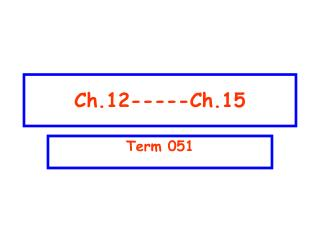DownloadDownload PresentationCh.12-----Ch.15

# Ch.12-----Ch.15

Télécharger la présentation## Ch.12-----Ch.15

- - - - - - - - - - - - - - - - - - - - - - - - - - - E N D - - - - - - - - - - - - - - - - - - - - - - - - - - -
##### Presentation Transcript

1. Ch.12-----Ch.15 Term 051

2. T051: Q: 16 . A cube of volume 8.0 cm3 is made of material with a bulk modulus of 3.5×109 N/m2. When it is subjected to a pressure of 3.0 × 105 Pa, the change in its volume (V) is(Ans: 6.9 x 10-4 cm3) Q: 17. A uniform rigid rod having a mass of 50 kg and a length of 2.0 m rests on two supports A and B as shown in the Fig. 3. When a block of mass 60 kg is kept at point C at a distance of x from the center, the rod is about to be lifted from A The value of x is: (Ans: 0.92 m ) Q: 18. A uniform beam having a mass of 60 kg and a length of 2.8 m is held in place at its lower end by a pin (P). Its upper end leans against a vertical frictionless wall as shown in the Fig. 4. What is the magnitude of the force by the pin on the beam? (Ans: 706 N) Ch-12

3. T051 Q#19: Calculate the magnitude and direction of net gravitational force on particle of mass m due to two particles each of mass M, where m =1000 kg and M =10000 kg and are arranged as shown in the Fig. 5.(Ans: 4.3 x 10-5 N directed towards negative x-axis) Q#20: One of the moons of planet Mars completes one revolution around Mars in 1.26 days. If the distance between Mars and the moon is 23460 km, calculate the mass of Mars.( Ans: 6.45 x 1023 kg) Q#21: A satellite of is in a circular orbit around a planet. If the kinetic energy of the satellite is 1.87 x 109 J, what is the mechanical energy of the satellite? (Ans: - 1.87 x 109 J) Q#22: A projectile was fired straight upward from Earth's surface with an initial speed vi such that it reaches a maximum height of 2RE above the Earth surface (take g = 9.80 m/s2 and RE = 6.37 x 106 m). The initial speed vi is (Ans: 10.5 km/s) Ch-13

4. T051: Q#23: Several cans of different sizes and shapes are all filled with the same liquid to the same height h (See Fig. 6). Then: (Ans: the pressure on the bottom of each can is the same) Q#24: Fig. 7 shows a U-tube with cross-sectional area A and partially filled with oil of density . A solid cylinder, which fits the tube tightly but can slide without friction, is placed in the right arm. The system is in equilibrium. The weight of the cylinder is: (Ans: ALg) Q#25: An object hangs from a spring balance The balance indicates 30N in air and 20N when the object is submerged in water. What does the balance indicate when the object is submersed in a liquid with a density that is half that of water? (Ans:25N) Q#26: An incompressible liquid flows along the pipe as shown in Fig. 8 with A1=2A2. The ratio of the mass flow rate R2/R1 is: (Ans: 1) Ch-14

5. T051 Q#27: In simple harmonic motion, the magnitude of the acceleration is greatest when: (Ans: the displacement is maximum) Q#28: A particle is in simple harmonic motion along the x axis. The amplitude of the motion is xm. At one point in its motion its kinetic energy is K = 5 J and its potential energy measured with U = 0 at x = 0) is U = 3 J. When it is at x = xm, the kinetic and potential energies are: (Ans: K = 0 and U = 8J) Q#29: A 0.25-kg block oscillates at the end of the spring with a spring constant of 200N/m. If the system has an energy of 6.0 J, then the amplitude of the oscillation is: (Ans: 0.24m) Q#30: A simple pendulum has length L and period T. As it passes through its equilibrium position, the string is suddenly clamped at its midpoint, its new period is (See Fig. 9). (Ans: T/2) Ch-15